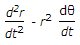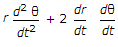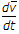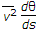# Civil Engineering - UPSC Civil Service Exam Questions

36.

In a compression member, plate element may buckle locally before the entire member fails. To avoid this, which of the following recommendations are made ?
1. Thickness of members is taken in terms of lengths of compression members
2. Length of element is increased
3. Length of member is increased
4. Outstand is decreased
Select the correct answer using the codes given below:

 A. 1, 2 and 3 B. 1, 3 and 4 C. 2 and 3 D. 1 and 4

Explanation:

No answer description available for this question. Let us discuss.

37.

The deflection at the free end of a cantilever of rectangular cross-section due to certain loading of 0.8 cm. If the depth of the section is doubled keeping the width the same, then the deflection at the free end due to the same loading will be

 A. 0.1 cm B. 0.4 cm C. 0.8 cm D. 1.6 cm

Explanation:

No answer description available for this question. Let us discuss.

38.

Self-purification of running streams may be due to

 A. sedimentation, oxidation land coagulation B. dilution, sedimentation and oxidation C. dilution, sedimentation and coagulation D. dilution, oxidation and coagulation

Explanation:

No answer description available for this question. Let us discuss.

39.

At a point in a strained material, if two mutually perpendicular tensile stresses of 2000 kg/cm2 and 1000 kg/cm2 are acting, then the intensity of tangential stress on a plane inclined at 15° to the axis of the minor stress will be :

 A. 125 kg/cm2 B. 250 kg/cm2 C. 500 kg/cm2 D. 1000 kg/cm2

Explanation:

No answer description available for this question. Let us discuss.

40.

A particle is moving on a plane curve with velocity v. 's' is the arc length of the particle from a fixed point on the curve and (r, θ) its position coordinates at time 't'. The transverse component of the acceleration of the particle is given by

 A.B.C.D.